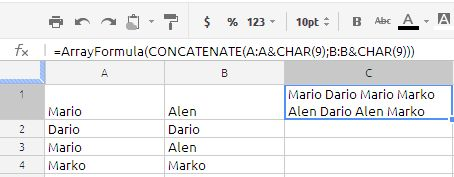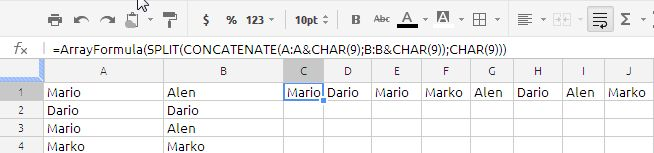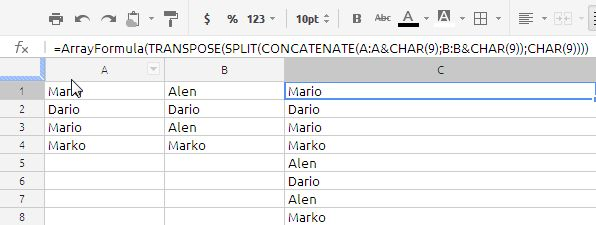## Extracting unique records from two columns

I have two columns:

A              B
Mario       Alen
Dario       Dario
Mario       Alen
Marko    Marko

I want in column C get only unique names from A and B
If I use =UNIQUE this will again create C and D columns, but I need it all in one column. How to do it?

C would look like this:

C
Mario
Dario
Marko
Alen

Solution:
If we try to do this using excel it will ugly big array formula, but the same can be achieved in google docs spread sheets without much fuss and very easy to understand.

I have tried with various formula but i could able to figure it out. Adam Lusk  Top Contributor in the Forum has came up with the following solution.
=ArrayFormula(UNIQUE(TRANSPOSE(SPLIT(CONCATENATE(A:A&CHAR(9);B:B&CHAR(9));CHAR(9)))))

now let us breakup the formula and understand the logic in the solution
Step 1:
=ArrayFormula(CONCATENATE(A:A&CHAR(9);B:B&CHAR(9))the whole data in cell A1 to B4 has been converted into text in the single cell separated by special character char(9) which is nothing but horizontal tabulation.

Step 2:
=ArrayFormula(SPLIT(CONCATENATE(A:A&CHAR(9);B:B&CHAR(9));CHAR(9)))in the above picture we have divided the single cell text to multiple cells using split function.

Step 3:
=ArrayFormula(TRANSPOSE(SPLIT(CONCATENATE(A:A&CHAR(9);B:B&CHAR(9));CHAR(9))))we have transposed the horizontal array into vertical array by using the Transpose function. the result will as show in the above picture.

Step 4:
=ArrayFormula(UNIQUE(TRANSPOSE(SPLIT(CONCATENATE(A:A&CHAR(9);B:B&CHAR(9));CHAR(9)))))in the final step we will get required results using the unique function in Google doc.

i would like thank Mr Adam Lusk for the contribution